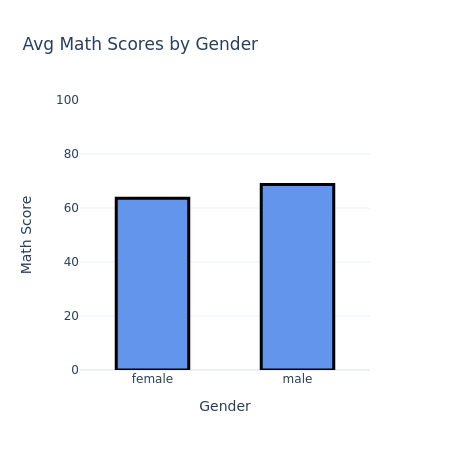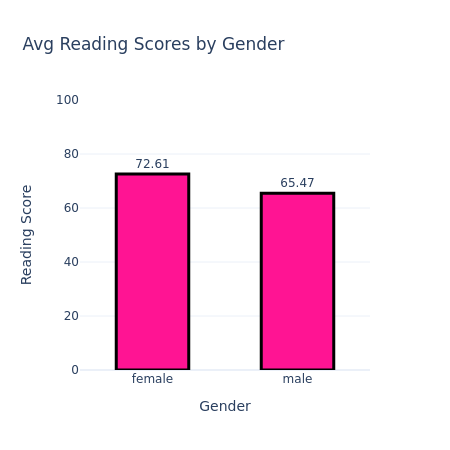# Plotly Tutorial

Sep 13, 2021

Plotly Tutorial 📊📈

What is Plotly?

Plotly is an interactive, open-source plotting library that supports over 40 unique chart types covering a wide range of statistical, financial, geographic, scientific, and 3-dimensional use-cases. There are three main elements of the visualizations that built with Plotly:

• Data: Specifies the type of visualization and the axes variables of it.
• Layout: Title, axis labels, font types, axes ranges, etc.
• Figure: Visualization may be completed by executing data and layout features in figure.

Scatter Plots

Scatter Plots

• Scatter plots allow the comparison of two variables for a set of data.
• Depending on the trend of scatter points, we could interpret a correlation.
• We can create scatter plots with go.Scatter
• Set the title of graph by using title in layout.
• The x and y parameters inside the title dictionary represent the position of the title.
• We can also specify the size of output with width and height
• We have to put the data and layout parts we filled in into the figure we created with go.Figure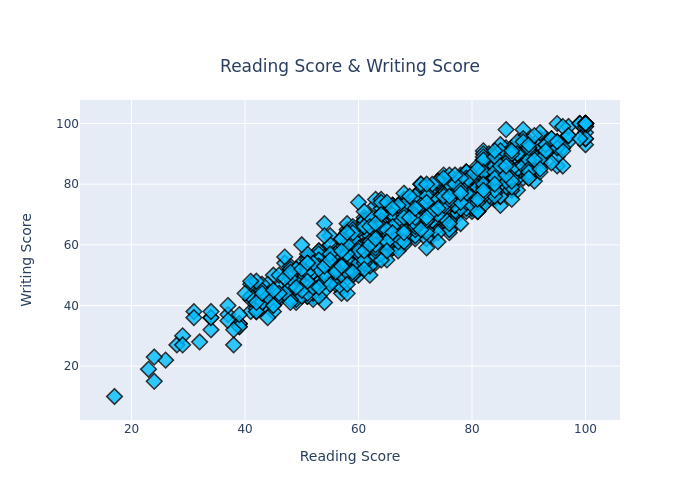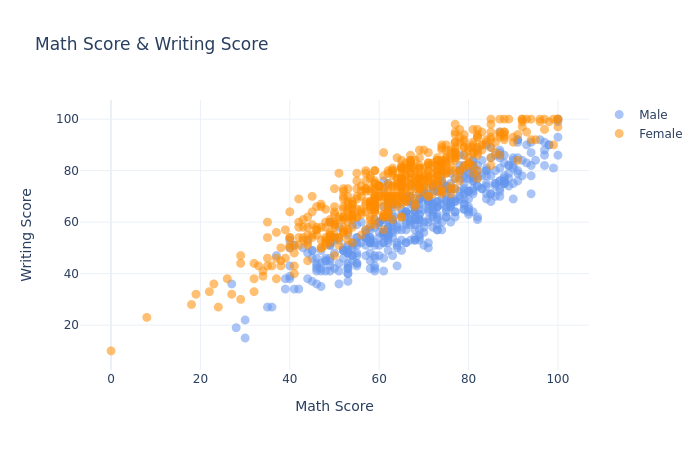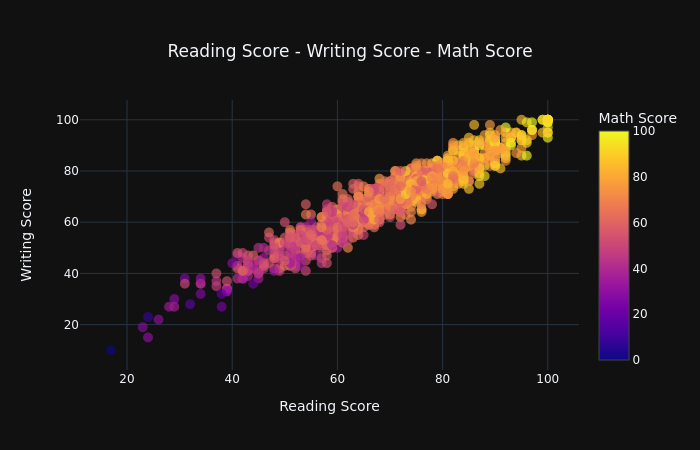Scatter Plots by Using For Loop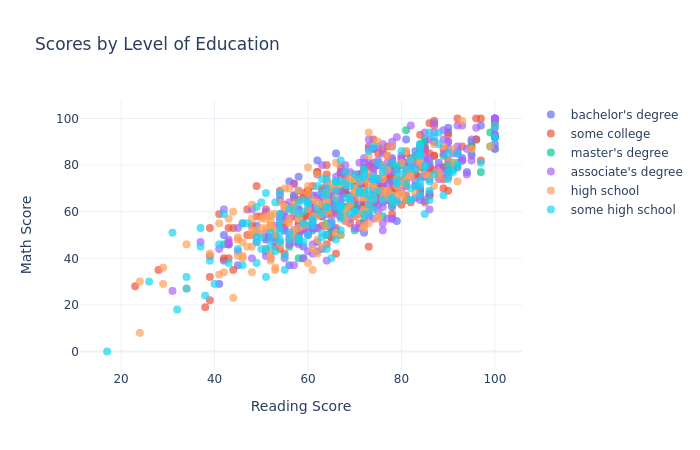Bubble Chart

Bubble Charts

• A bubble chart is a type of chart that displays three dimensions of data.
• Bubble charts can be considered a variation of the scatter plot, in which the data points are replaced with bubbles.
• Define the third variable with size in bubble charts.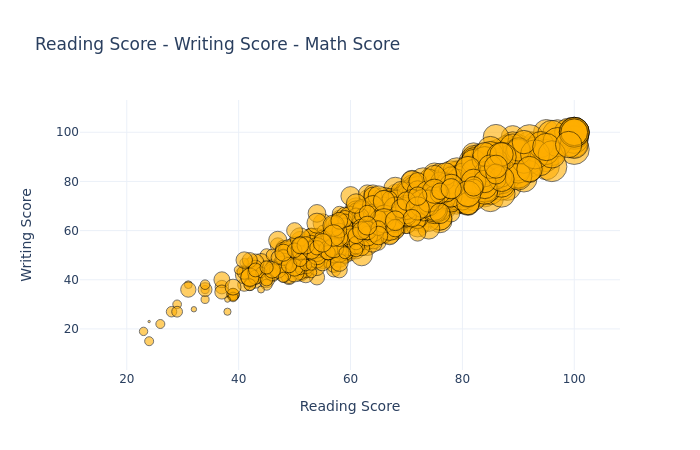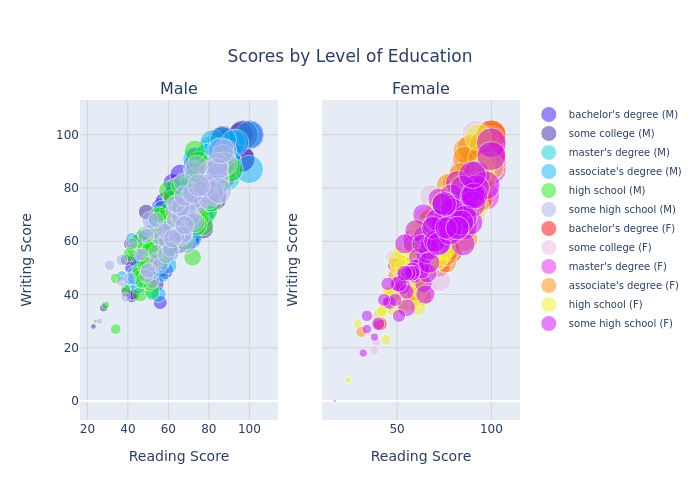3D Bubble Chart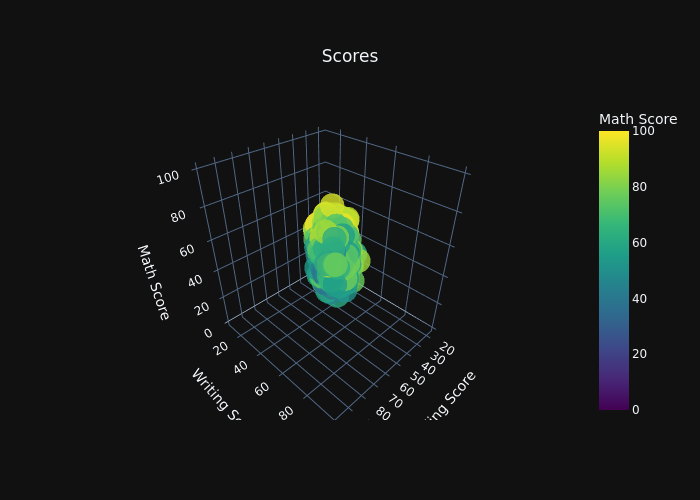Line Chart

Line Charts

• A line chart displays a series of data points (markers) connected by line segments.
• It is similar to a scatter plot except that the measurement points are ordered (typically by their x-axis value) and joined with straight line segments.
• Often used to visualize a trend in data over intervals of time - known as a time series.
• To create a line chart, tune the mode parameter as “line”.
• Like scatter plots, you can edit marker qualities like line color,line width, etc. in line parameter.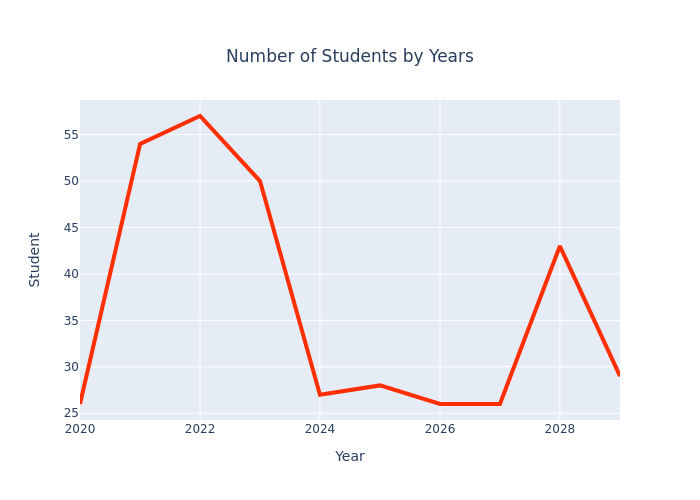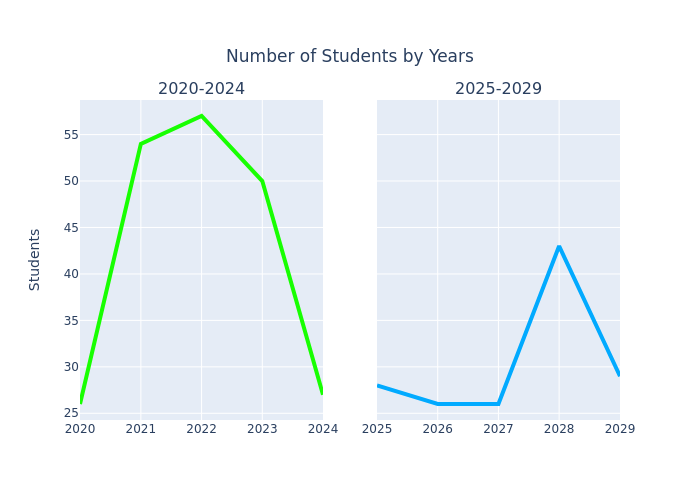Bar Chart

Bar Charts

• A bar chart presents categorical data with rectangular bars with heights (or lengths) proportional to the values that they represent.
• Built a bar chart with go.Bar
• Use text to display values on each bar.
• Bars can be edited with marker